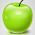Aptitude: Percentage Problem Tricks for Competitive Exams - TNPSC GURU - TNPSC Group 2A Results, cut offMark - TNPSC

## Friday, April 25

Percentages
A good thing about percentage is that you don't need to learn any difficult formulas. For most of the problems you need to do a simple multiplication to get the result.

Very few problems are asked directly from Percentage type.
For example: Let A=50, B=100. What percentage is A of B ?

Usually the percentage based problems are asked from the Profit - Loss, Distance - Time and other Sections (These topics will be covered in our upcoming tutorials).
For example: A table is sold for Rs. 414 at a profit of 15%. How much did it cost?[TNPSC Group 2 2013 Question]
So it is also important to have a little knowledge about these sections too to solve a percentage based problem without any confusions.

Percentage in simple terms is the number out of 100 or value per 100.

Type 1: Finding the percentage of one item from a number of Items
In a class room of 150 students, 50 students were present. find the percentage of students present and absent respectively?
To find the percentage, keep the total number in the denominator and the required number in the numerator and multiply it with 100.

In this problem
Percentage = (Numerator/Denominator)x100 = (required number/total number)x 100
Percentage of Students present = (50/150) x 100 = 33.33%
Percentage of Students Absent = (100/150) x 100 = 66.66%
Do it yourself Problems:
1. An Automobile company produces both two wheeler and Four Wheeler vehicles. The number of two wheeler produced in one month is 320 out of the total 1000 vehicles. Find the percentage of number of Four Wheeler vehicles produced?
Type 2: Finding the Number or Value from the number of Items for the given Percentage.
In a class room of 160 students, 75% of the students were present. find the number of students present and absent respectively?
Number = (required percentage / 100) x Total Number
Number of Students Present = (75/100) x 160 = 120
Number of Students Absent = (25/100) x 160 = 40

#### 1 comment:

1.good for beginers like me...thanks...

Post your feedback and doubts in the comment box below.

Thanks for visiting our Website..